## How to Calculate and Solve for the Area, Length, Width and Height of a Cuboid | Nickzom Calculator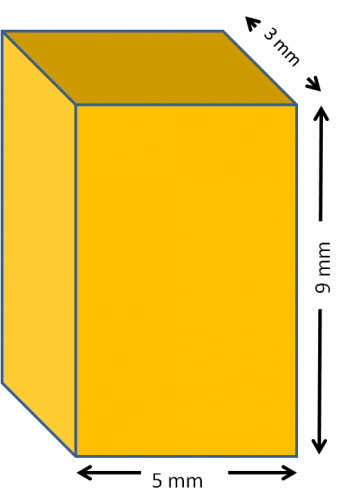The image above represents a cuboid.
To compute the area of a cuboid requires three essential parameters which are the length, width and height of the cuboid.

The formula for computing the area of a cuboid is:

A = 2(lw + lh + wh)

Where:
A = Area of the Cuboid
l = Length of the Cuboid
w = Width of the Cuboid
h = Height of the Cuboid

Let’s solve an example
Find the area of a cuboid with a length of 5 cm, width of 3 cm and a height of 9 cm.

This implies that:
l = length of the cuboid = 5
w = width of the cuboid = 3
h= = height of the cuboid = 9

A = 2(lw + lh + wh)
A = 2(5 x 3 + 5 x 9 + 3 x 9)
A = 2(87)
A = 174

Therefore, the area of the cuboid is 174 cm2.

Calculating the Length of a cuboid when Area, Width and Height are Given

The formula is l = A – 2(w)(h) / 2(w) + 2(h)

Where;
A = Area of the Cuboid
l = Length of the Cuboid
w = Width of the Cuboid
h = Height of the Cuboid

Let’s solve an example:
Find the length of a cuboid with an area of 140 cm2 , a width of 4 cm and a height of 12 cm

This implies that;
A = Area of the cuboid = 140 cm2
w = width of the cuboid = 4 cm
h  = height of the cuboid = 12 cm

l =  A – 2(w)(h) / 2(w) + 2(h)
l = 140 – 2(4)(12) / 2(4) + 2(12)
l = 140 – 96 / 8 + 24
l = 44 / 32
l = 1.375 cm

Therefore, the length of the cuboid is 1.375 cm.

Calculating the Width of a cuboid when Area, Length and Height are Given

The formula is w = A – 2(l)(h) / 2(l) + 2(h)

Where;
A = Area of the Cuboid
l = Length of the Cuboid
w = Width of the Cuboid
h = Height of the Cuboid

Let’s solve an example:
Find the width of a cuboid with an area of 200 cm2 , a length of 5 cm and a height of 12 cm

This implies that;
A = Area of the cuboid = 200 cm2
l = length of the cuboid = 5 cm
h  = height of the cuboid = 12 cm

w =  A – 2(l)(h) / 2(l) + 2(h)
w = 200 – 2(5)(12) / 2(5) + 2(12)
w = 200 – 120 / 10 + 24
w = 80 / 34
w = 2.353 cm

Therefore, the width of the cuboid is 2.353 cm.

Calculating the Height of a cuboid when Area, Length and Width are Given

The formula is h = A – 2(l)(w) / 2(l) + 2(w)

Where;
A = Area of the Cuboid
l = Length of the Cuboid
w = Width of the Cuboid
h = Height of the Cuboid

Let’s solve an example:
Find the height of a cuboid with an area of 300 cm2 , a length of 6 cm and a width of 2 cm

This implies that;
A = Area of the cuboid = 300 cm2
l = length of the cuboid = 6 cm
w  = width of the cuboid = 2 cm

h =  A – 2(l)(w) / 2(l) + 2(w)
h = 300 – 2(6)(2) / 2(6) + 2(2)
h = 300 – 24 / 12 + 4
h = 276 / 16
h = 17.25 cm

Therefore, the height of the cuboid is 17.25 cm.

## How to Calculate and Solve for the Length of the Diagonal and Area of a Rhombus | The Calculator Encyclopedia

The above image is a rhombus.

To compute the area of a rhombus, two essential parameters are needed and this parameters are length of the diagonal (p) and length of the diagonal (q).

The formula for calculating the area of a rhombus:

A = pq ⁄ 2

Where;
A = Area of the rhombus
p = length of the diagonal
q = length of the diagonal

Let’s solve an example;
Find the area of a rhombus when the length of the diagonal (p) is 10 cm and the length of the diagonal (q) is 18 cm.

This implies that;
p = length of the diagonal = 10 cm
q = length of the diagonal = 18 cm

A = pq2
A = 10 x 18 / 2
A = 180 / 2
A = 90

Therefore, the area of the rhombus is 90 cm2.

Calculating the length of the diagonal (p) using Area of the Rhombus and length of the diagonal (q).

p = 2A / q

Where;
A = Area of the rhombus
q = length of the diagonal

Let’s solve an example;
Given that the length of the diagonal (q) is 20 cm with an area of 60 cm2. Find the length of the diagonal (p)?

This implies that;
A = Area of the rhombus = 60 cm2
q = length of the diagonal = 20 cm

p = 2A / q
p = 2 x 60 / 20
p = 120 / 20
p = 6

Therefore, the length of the diagonal is 6 cm.

## How to Calculate and Solve for the Area and Length of a Parallelogram | The Calculator EncyclopediaThe image above represents a parallelogram.
To compute the area of a parallelogram requires two essential parameters which are the base and height of the parallelogram.

The formula for computing the area of a parallelogram is:

A = b x h

Where:
A = Area of a parallelogram
b = base of the parallelogram
h = height of the parallelogram

Let’s solve an example
Find the area of a parallelogram with a base of 12 cm and a height of 4 cm.

This implies that:
b = base of the parallelogram = 12
h = height of the parallelogram = 4

A = b x h
A = 12 x 4
A = 48

Therefore, the area of the parallelogram is 48 cm2.

Calculating the base of a parallelogram when Area and Height is Given

The formula is b = A / h

Where;
A = Area of a parallelogram
b = base of the parallelogram
h = height of the parallelogram

Let’s solve an example:
Find the base of a parallelogram with an area of 40 cm² and a height of 8 cm

This implies that;
A = Area of the parallelogram = 40 cm²
h = height of the parallelogram = 8 cm

b = A / h
b = 40 / 8
b = 5

Therefore, the base of the parallelogram is 5 cm.

Calculating the height of a parallelogram when Area and Base is Given

The formula is h = A / b

Where;
A = Area of a parallelogram
b = base of the parallelogram
h = height of the parallelogram

Let’s solve an example:
Find the height of a parallelogram with an area of 60 cm² and a base of 6 cm

This implies that;
A = Area of the parallelogram = 60 cm²
b = base of the parallelogram = 6 cm

h = A / b
h = 60 / 6
h = 10

Therefore, the height of the parallelogram is 10 cm.

## How to Calculate and Solve for the Volume and Length of a Cube | The Calculator Encyclopedia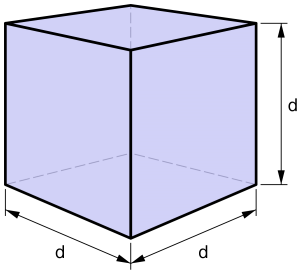The image above is a cube.

To compute the volume of a cube, one essential parameter is needed and this parameter is the length of the cube (l).

The formula for calculating the volume of a cube is;

V = l3

Where;

V = Volume of a cube
l = Length of a cube

Let’s solve an example:
Find the volume of a cube where the length of a cube is 4 cm.

This implies that;
l = length of the cube = 4 cm.

V = l3
V = 43
V = 64 cm3

Therefore, the volume of the cube is 64 cm3

Calculating the Length of a cube using the Volume of the cube.

The formula is l = 3√V

Where;

V = Volume of a cube
l = length of a cube

Let’s solve an example:
Find the length of a cube where the volume of the cube is 120 cm3

This implies that;
V = Volume of the cube = 120 cm3

l = 3√V
l = 3√120
l = 4.93

Therefore, the length of the cube is 4.93 cm.

## How to Calculate and Solve for the Perimeter, Length and Width of a Rectangle | The Calculator EncyclopediaThe image above is a rectangle with a length of 8 cm and a width of 5 cm.
To compute the perimeter of a rectangle requires two parameters which are the length of the rectangle and the width of the rectangle.

The formula for calculating the perimeter of a rectangle is:

P = 2 (l + w)

Where:

P = Perimeter of a Rectangle
l = Length of a Rectangle
w = Width of a Rectangle

Let’s solve an example:
Find the perimeter of a rectangle where the length of the rectangle is 8 cm and the width of the rectangle is 5 cm.

This implies that:
l = Length of the Rectangle = 8 cm
w = Width of the Rectangle = 5 cm

P = 2 (l + w)
P = 2 (8 + 5)
P = 2 (13)
P = 26

Therefore, the perimeter of the rectangle is 26 cm.

How to Calculate the Length of a Rectangle when the Perimeter of the Rectangle and the Width of the Rectangle is Given

l = P / 2 – w

Where:
l = Length of the Rectangle
P = Perimeter of the Rectangle
w = Width of the Rectangle

## How to Calculate and Solve for the Area, Length and Height of a Trapezium | The Calculator Encyclopedia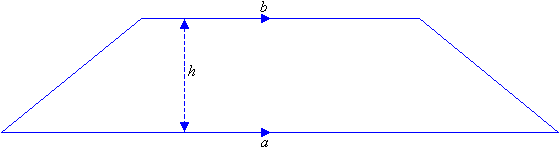The image above is a Trapezium

To compute the area of a trapezium, three essential parameter is needed and they are the length of the top side (a), length of the bottom side (b) and the height of the trapezium (h).

The formula for calculating the area of a trapezium is;

A = 0.5[a + b]h

Where;
A = Area of a trapezium
a = length of the top side of the trapezium
b = length of the bottom side of the trapezium
h = height of the trapezium

Let’s solve an example;
Find the area of a trapezium where length of top side (a) is 7 cm, length of bottom side (b) is 12 cm and height of trapezium (h) is 10 cm.

This implies that;
a = Length of top side of the trapezium = 7 cm
b = Length of bottom side of the trapezium = 12 cm
h = Height of the trapezium = 10 cm

A = 0.5[a + b]h
A = 0.5[7 + 12]10
A = 0.510
A = 95

Therefore, the area of a trapezium is 95 cm2

How to Calculate the Height of a Trapezium when the Area, Length of top side and Length of bottom side of the Trapezium is given.

The formula is h = A / 0.5(a + b)

Where;
A = Area of the trapezium
a = Length of the top side of the trapezium
b = Length of the bottom side of the trapezium

Let’s solve for an example;
Given that the length of top side (a) is 10 cm, length of bottom side (b) is 14 cm and the area of the trapezium is 20 cm2 Find the height of the trapezium?

This implies that;

A = Area of the trapezium = 20 cm2
a = Length of top side of the trapezium = 10 cm
b = Length of bottom side of the trapezium = 14 cm

h = A / 0.5(a + b)
h = 20 / 0.5(10 + 14)
h = 20 / 0.5(24)
h = 20 / 12
h = 1.667

Therefore, the height of the trapezium is 1.667 cm.

## How to Calculate and Solve for the Perimeter and Length of a Square | The Calculator Encyclopedia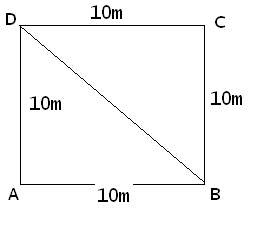The image above represents a square.
To compute the perimeter of a square requires one essential parameter which is the length of one side of a square.

The formula for computing the perimeter of a square is:

P = 4 x l

Where:
P = Perimeter of a Square
l = Length of one side of a Square

Let’s solve an example
Find the perimeter of a square which has a length of 10 m.

This implies that:
l = length of one side of a square = 10

P = 4 x l
P = 4 x 10
P = 40

Therefore, the perimeter of the square is 40 m.

Calculating the Length of a Square when Perimeter is Given

The formula is l = p / 4

Where;
P = Perimeter of a square
l = Length of a square

Let’s solve an example:
Find the length of a square where the perimeter of a square is 100 m.

This implies that;
P = Perimeter of a square = 100 m

l = p / 4
l = 100 / 4
l = 25

Therefore, the length of the square is 25 m.

## How to Calculate and Solve for the Area, Width and Length of a Rectangle | The Calculator Encyclopedia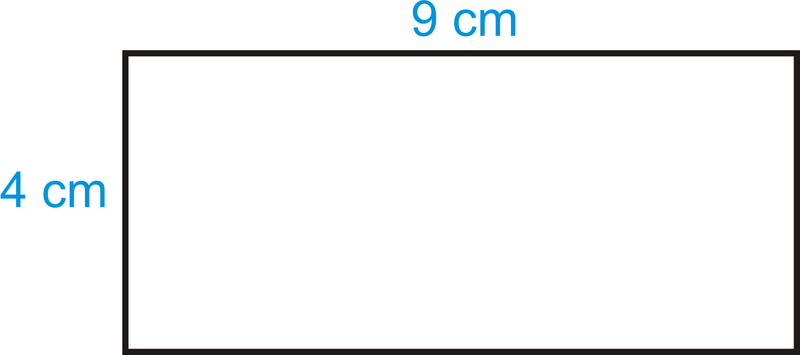The image above is a rectangle with a length of 9 cm and a width of 4 cm.
To compute the area of a rectangle requires two essential parameters which are the length of the rectangle and the width of the rectangle.

The formula for calculating the area of a rectangle is:

A = lw

Where:

A = Area of a Rectangle
l = Length of a Rectangle
w = Width of a Rectangle

Let’s solve an example
Find the area of a rectangle where the length of the rectangle is 9 cm and the width of the rectangle is 4 cm.

This implies that:
l = Length of the Rectangle = 9 cm
w = Width of the Rectangle = 4 cm

A = lw
A = (9)(4)
A = 36

Therefore, the area of the rectangle is 36 cm2.

How to Calculate the Length of a Rectangle when the Area and Width of the Rectangle is Given

The formula for calculating the length of the rectangle is:

l = A / w

Where:

l = Length of the Rectangle
A = Area of the Rectangle
w = Width of the Rectangle

Let’s solve an example:
Find the length of a rectangle where the area of the rectangle is 45 cm2 and the width of the rectangle is 9 cm.

This implies that:
A = Area of the Rectangle = 45 cm2
w = Width of the Rectangle = 9 cm

l = A / w
l = 45 / 9
l = 5

Therefore, the length of the rectangle is 5 cm.

How to Calculate the Width of a Rectangle when the Area and Length of the Rectangle is Given

The formula for calculating the width of the rectangle is:

w = A / l

Where:

w = Width of the Rectangle
A = Area of the Rectangle
l = Length of the Rectangle

## How to Calculate and Solve for the Area and Length of a Square | The Calculator Encyclopedia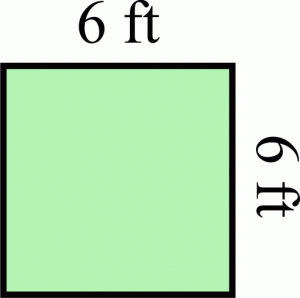The image above represents a square.
To compute the area of a square requires one essential parameter which is the length of one side of a square.

The formula for computing the area of a square is:

A = l2

Where:
A = Area of a Square
l = Length of one side of a Square

Let’s solve an example
Find the area of a square which has a length of 6 ft.

This implies that:
l = length of one side of a square = 6

A = l2
A = 62
A = 6 x 6
A = 36

Therefore, the area of the square is 36 ft2.

Calculating the Length of a Square when Area is Given

The formula is l = √[A]

Where;
A = Area of a square
l = Length of a square

Let’s solve an example:
Find the length of a square where the area of a square is 81 ft2.

This implies that;
A = Area of a square = 81 ft2

l = √[A]
l = √
l = 9

Therefore, the length of the square is 9 ft.

## How to Calculate and Solve for the Centre of Gravity of a Cube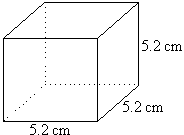The image above is a cube with a length of 5.2 cm.
To compute the centre of gravity of a cube, one essential parameter is needed and this parameter is the length of the cube (l).

The formula for calculating the centre of gravity of a cube is:

C.G. = 0.5(l)

Where:
l = Length of the Cube
C.G. = Centre of Gravity

Let’s solve an example:
Find the centre of gravity of a cube where the length of a side of the cube is 5.2 cm.

This implies that:
l = Length of the Cube = 5.2